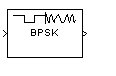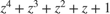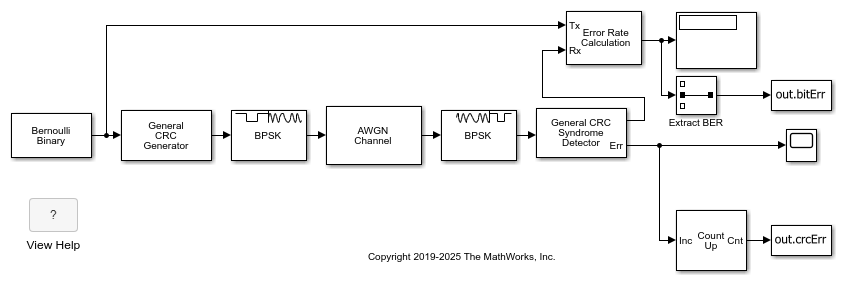# BPSK Modulator Baseband

Modulate using BPSK method

•Libraries:
Communications Toolbox / Modulation / Digital Baseband Modulation / PM
Communications Toolbox HDL Support / Modulation / PM

## Description

The BPSK Modulator Baseband block modulates a signal by using the binary phase shift keying (BPSK) method. The output is a baseband representation of the modulated signal. The input signal must be a discrete-time binary-valued signal. If the input bit is 0 or 1, then the modulated symbol is exp(jθ) or -exp(jθ), respectively. The ```Phase offset (rad)``` parameter specifies the value of θ in radians.

## Examples

expand all

Use a CRC code to detect frame errors in a noisy BPSK signal.

In the `cm_ex_crc_noisy_bpsk_frames` model, the CRC generator and detector pair use a standard CRC-4 polynomial,. The length of the CRC is 4 bits as determined by the degree of the polynomial. The number of checksums per frame is 1, so the full transmission frame has one CRC appended at the end.

A binary signal frame gets a CRC code appended to the end of the frame. BPSK modulation is applied to the signal and the signal passes through an AWGN channel. The signal is demodulated, and then a CRC syndrome detector removes the CRC and calculates the CRC errors.Generate 12-bit frames of binary data and append CRC bits. Based on the degree of the polynomial, 4 bits are appended to each frame. Apply BPSK modulation and pass the signal through an AWGN channel. Demodulate and use the CRC detector to determine if the frame is in error.The results of the CRC detection are compared to a BER calculation.

```Number of bit errors detected: 6 Number of crc errors detected: 7 ```

## Ports

### Input

expand all

Input signal, specified as a scalar or vector with element values in the range [0,M – 1], where M is the modulation order. If you specify a binary vector, the number of elements must be an integer multiple of the number of bits per symbol. The number of bits per symbol is equal to log2(M).

Data Types: `single` | `double` | `int8` | `int16` | `int32` | `uint8` | `uint16` | `uint32` | `Boolean`
Complex Number Support: Yes

### Output

expand all

BPSK-modulated baseband signal, returned as a complex-valued scalar or vector.

Data Types: `single` | `double` | `fixed point`

## Parameters

expand all

To edit block parameters interactively, use the Property Inspector. From the Simulink® Toolstrip, on the Simulation tab, in the Prepare gallery, select .

### Main

Phase offset of the zeroth point of the constellation in radians, specified as a scalar.

Example: `pi/4`

Click on the block mask to visualize a signal constellation for the specified block parameters. Before viewing a constellation, apply the parameter settings. For more information, see View Constellation of Modulator Block.

### Data Types

The output data type, specified as `double`, `single`, `fixdt(1,16)`, ```fixdt(1,16, 0)```, ```Inherit via back propagation```, or ```<data type expression>```.

Setting this parameter to

• `fixdt(1,16)`, `fixdt(1,16.0)`, or `<data type expression>` enables parameters in which you can further specify details.

• `Inherit via back propagation` sets the output data type and scaling to match the following block.

The Data Type Assistant helps you set data attributes. To use the Data Type Assistant, click. For more information, see Specify Data Types Using Data Type Assistant (Simulink).

## Block Characteristics

 Data Types `Boolean` | `double` | `fixed pointa, b` | `integer` | `single` Multidimensional Signals `no` Variable-Size Signals `yes` a ufix(ceil(log2(M))) only at the input for M-ary modulation.b Fixed-point outputs must be signed.

## Algorithms

Phase modulation is a linear baseband modulation technique in which the message modulates the phase of a constant amplitude signal. Binary Phase Shift Keying (BPSK) is a two phase modulation scheme, where the 0’s and 1’s in a binary message are represented by two different phase states in the carrier signal

`${s}_{n}\left(t\right)=\sqrt{\frac{2{E}_{b}}{{T}_{b}}}\mathrm{cos}\left(2\pi {f}_{c}t+{\varphi }_{n}\right),$`

for where:

• ϕn = πm, m∈{0,1}.

• Eb is the energy per bit.

• Tb is the bit duration.

• fc is the carrier frequency.

In MATLAB®, the baseband representation of a BPSK signal is

`${s}_{n}\left(t\right)={e}^{-i{\varphi }_{n}}=\mathrm{cos}\left(\pi n\right).$`

The BPSK signal has two phases: 0 and π. The probability of a bit error in an AWGN channel is

`${P}_{b}=Q\left(\sqrt{\frac{2{E}_{b}}{{N}_{0}}}\right),$`

where N0 is the noise power spectral density.

## Version History

Introduced before R2006a